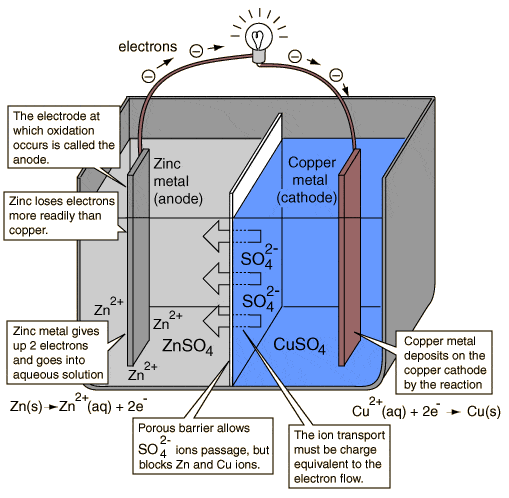# Electrostatic Potential: Value at zero distance from charge

• Prateek Kumar Jain

#### Prateek Kumar Jain

Formula for Electrostatic Potential due to a point charge is V=1/4π∈ Q1 Q2/r

This implies that at r=0 value of the potential should be infinity.

Is it True.

If that is the case then how we say the terminals of a battery having positive and negative charge are having definite value of potentials. I think as per the formula potential of terminals of battery should also be infinity?

Battery terminals are not points. When you get close enough to a "point" charge, classical theory breaks down and you need quantum theory.

•Prateek Kumar Jain
Battery terminals are not points. When you get close enough to a "point" charge, classical theory breaks down and you need quantum theory.

I understand Battery terminals are not points. But they create a driving force for electrons to move in an Electric Circuit.If you see the above figure copied from "http://hyperphysics.phy-astr.gsu.edu/hbase/chemical/electrochem.html". I don't understand how potential is created in the battery terminals?

Potential is created by charges. Here we have Zn Charge and Cu Charge, How potential develops in the electrodes?

I think as per the formula potential of terminals of battery should also be infinity
In physics you cannot just blindly go plugging numbers into formulas. You need to know the limitations or approximations or assumptions of a formula.

Coulomb's law is limited to the outside of a spherically symmetric charge distribution. The terminals of a battery are not spherically symmetric, and even if they were their radius would be finite.

•Prateek Kumar Jain